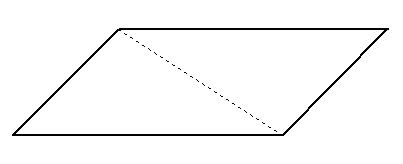SEARCH HOMEMath Central Quandaries & QueriesSubject: geometry Name: jenniffeir Who are you: Student Can a parallelogram have two 45 degree angles and two 75 degree angles?Hi Jenniffeir,

Think about this: If you draw one of the parallelogram's diagonals, you will always get two triangles:What is the sum of the interior angles of a triangle? So what should the sum of two triangles be? So, what should the interior angles of a parallelogram add to?

HaleyMath Central is supported by the University of Regina and The Pacific Institute for the Mathematical Sciences.